TitleCollege Algebra
Tutorial 42:
Exponential FunctionsLearning Objectives

 After completing this tutorial, you should be able to: Use the exponent and e keys on your calculator. Evaluate an exponential function. Graph exponential functions. Calculate compound interest problems.Introduction

 In this tutorial we will be looking at exponential functions.  It is good to be familiar with exponents in general before you start this lesson.  What is different in this tutorial is that your variable x is now your exponent, where before, the variable x was in your base.   I can’t stress this enough,  if you are not comfortable with the basics of exponents  you need to review exponents which you can find in  Tutorial 2: Integer Exponents.  One thing we will be looking at in this section is using your exponent and e keys on your calculator.  So make sure that you have your calculator ready to go.  It will also help you with the graphing problems.  Let's say we take a look at these exponential functions.Tutorial

 Definition of Exponential Function   The function f defined by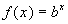where b > 0, b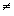1, and the exponent x is any real number, is called an exponential function.

 Again, note that the variable x is in the exponent as opposed to the base when we are dealing with an exponential functions.  Also note that in this definition, the base b is restricted to being a positive number other than 1.

 Exponent Key  on Calculator

Before we get going with the actual exponential functions, I wanted to make sure that everyone knew how to use the exponent key on their calculator.  Since there are a lot of different calculators I will be go over the more common ones.  At this point you need to check to make sure that you know how to use your exponent key, because we will be using it heavily throughout this as well as the next unit.

The two main exponent keys found on calculators are (you will only have one of these):

 Caret top key:     ^ Base raised to an exponent key: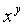OR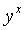Check to see if you have one of the two main type of exponent keys (you won’t have both).   If you don’t have one check the other.  If you don’t see either, look in the reference manual that came with the calculator to see which key it is.

 The caret top key is most frequently found on graphing calculators but can be found on other types of calculators. On most graphing calculators your exponent key is the caret top key:   ^.  If you have the caret top key let's practice taking 15 and raising  it to the 5th power.  To do this you would type in 15^5 and press your enter or  =  key.    If you got 759375, you entered it in correctly.  If not, try again. If you still can't get it look in your reference manual that came with the calculator.    The key that look likeORare most common in business and scientific calculators, but can be found on other types of calculators. On most business and scientific calculators, the exponent key looks likeoror very similar to this .  If you have this key let's practice taking 15 and raising  it to the 5th power.  In this situation, you first type in your base then you activate your exponent key and then you type in your exponent and then press enter or equal.  Go ahead and try it out by finding 15 raised to the 5th power.  Type in 15, then pressorand then type in 5 and press the enter or equal key.  You should have gotten 759375 as your answer.  If not, try again.  If you still can't get it look in your reference manual that came with the calculator.

 Base e e is approximately 2.718281828...

 The exponential function with base e is called the natural exponential function.  e has a value attached it (similar to pi). e is approximately 2.718281828...  This base is used in economic analysis and problems involving natural growth and decay.  At this point, we are just going to learn how to find the value of e raised to an exponent using the calculator.

 e Key  on Calculator

I want to make sure that everyone knows how to use the e key on their calculator.  Since there are a lot of different calculators I will be go over the more common ones.  At this point you need to check to make sure that you know how to use this key, because we will be using it heavily.

The two main e keys found on calculators are (you will only have one of these):

 e key and the Caret top key: (2 keys) e  and then  ^ e raised to an exponent key:  (1 key)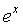Check to see if you have one of the two main type of e keys (you won’t have both).   If you don’t have one check the other.  If you don’t see either, look in the reference manual that came with the calculator to see which key it is.

 Having to use the e key with the caret top key is most frequently found on graphing calculators but can be found on other types of calculators. On most graphing calculators in order to raise e to a power you must press the e key first, then press your exponent key  ^, and then enter in your exponent.     If you have the e key (with no exponent showing) and the caret top key let's practice taking e and raising  it to the 5th power.  To do this you would press the e key,  then press the exponent key ^, and then type in 5.  It should look like this on your screen: e^5.    If you got 148.41316..., you entered it in correctly.  If not, try again. If you still can't get it look in your reference manual that came with the calculator.   The key that looks likeis most common in business and scientific calculators, but can be found on other types of calculators. On most business and scientific calculators, the e function key looks likeor very similar to this.  So check for this  key - note that the difference between this and the one above is that this key has a variable exponent showing on the key - the above key only has an e (no exponent.  If you have this key let's practice taking e and raising  it to the 5th power.  In this situation, you first type in your exponent and  then you activate yourkey.  Go ahead and try it out by finding e raised to the 5th power.  Type in 5 and then press.  You should have gotten 148.41316... as your answer.  If not, try again. If you still can't get it look in your reference manual that came with the calculator.

 Using the Calculator, in generalExample 1: Approximate the number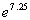using a calculator.  Round to four decimal places.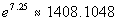Try this using your calculator and see if you get the answer that I got.  If you didn’t, refer back to e key on calculator that I have above.Example 2: Approximate the number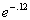using a calculator.  Round to four decimal places.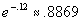Try this using your calculator and see if you get the answer that I got.  If you didn’t, refer back to e key on calculator that I have above.

 Graphing Exponential Functions

 Step 1: Find ordered pairs.

 I have found that the best way to do this is to do it the same each time.  In other words, put in the same values for x each time and then find it’s corresponding y value for the given function.

 Step 2: Plot points.

 This done exactly the same way you plotted points when you graphed lines and parabolas.

 Step 3: Draw curve.

 The basic curve of an exponential function looks like the following: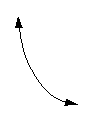OR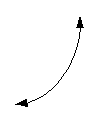Example 3:  Graph the function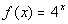.

 Note that the base = 4 and the exponent is our variable x.  Also note, that it is in the basic form given by the definition above,  in other words there are no other factors effecting this function.

 Step 1: Find ordered pairs.

I have found that the best way to do this is to do it the same each time.  In other words, put in the same values for x each time and then find it’s corresponding y value for the given function.

 x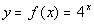(x, y) -2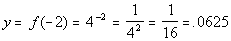(-2, .0625) -1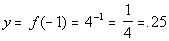(-1, .25) 0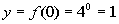(0, 1) 1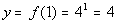(1, 4) 2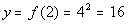(2, 16)

 Step 2: Plot points. AND Step 3: Draw curve.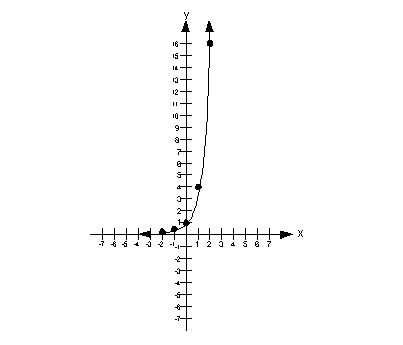Example 4:  Graph the function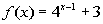.

 Note that the base = 4 and the exponent is  x - 1. There are two outside factors, we are subtracting 1 from our variable x in the exponent AND we are adding 3 to our base after we raise it to the exponent x - 1.

 Step 1: Find ordered pairs.

I have found that the best way to do this is to do it the same each time.  In other words, put in the same values for x each time and then find it’s corresponding y value for the given function.

 x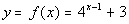(x, y) -2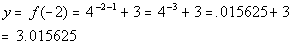(-2, 3.015625) -1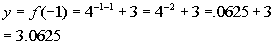(-1, 3.0625) 0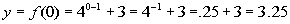(0, 3.25) 1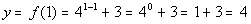(1, 4) 2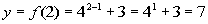(2, 7)

 Step 2: Plot points. AND Step 3: Draw curve.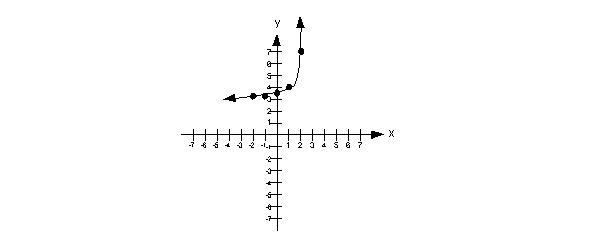Example 5:  Graph the function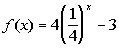.

 Note that the base = 1/4 and the exponent is our variable x. This time there are two outside factors,  we are multiplying our basic form by 4 and then we are subtracting 3.  Be careful that you use the order of operations when working a problem like this.  We need to deal with the exponent first BEFORE we multiply by 4.  It is really tempting to cross the 4 on the outside with the 4 in the denominator of our base.  But the 4 in the denominator is enclosed by a ( ) with an exponent attached to it, so we have to deal with that first before getting the 4 on the outside involved. Let’s find some order pairs. Again, we will use the same input values for x we used in examples 3 and 4 and find the corresponding output values for this exponential function.

 Step 1: Find ordered pairs.

I have found that the best way to do this is to do it the same each time.  In other words, put in the same values for x each time and then find it’s corresponding y value for the given function.

 x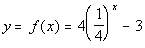(x, y) -2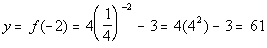(-2, 61) -1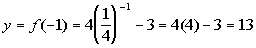(-1, 13) 0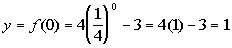(0, 1) 1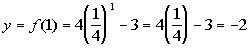(1, -2) 2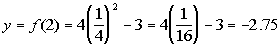(2, -2.75)

 Step 2: Plot points. AND Step 3: Draw curve.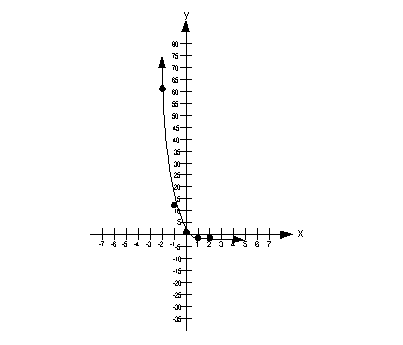Compound Amount and Interest

 Compound interest means that at the end of each interest period the interest earned for that period is added to the previous principle (the invested amount) so that, it too, earns interest over the next interest period.  In other words this is an accumulative interest.

 Compound Interest Formula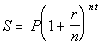where,  S = compound or accumulated amount  P = Principal   (starting value)  r = nominal rate (annual % rate)  n = the number of compound periods per year  t = number of years

 Note that this formula may look different than the one from your math book.  Sometimes an A is used for compound or accumulated interest instead of an S.  Also sometimes r represents a periodic rate where in the above formula it is a nominal rate.  In that type of  formula you would not see r being divided by n, but you would still need to do that if your r represents a periodic rate.  It just isn't shown as part of the formula. Just keep in mind that no matter how the formula looks the concept is the same.Example 6:   Find the a) compound amount AND b) the compound interest for the given investment and rate.  \$15000 for 14 years at an annual rate of 5% compounded monthly.

 Note that the steps shown in examples 6, 7, and 8 go with how to put it piece by piece into a business or scientific calculator.  If you have a graphing calculator, you can chose to do it this way (piece by piece) or you can plug the whole formula in and then press enter to get your final answer. Also note that different calculators round to different place values.   So when you are putting this into your calculator keep in mind that yours may be rounding to a different place than mine, so it may be slightly different for the last digit to the right of the decimal.  Also, don’t  round anything until you get to the final answer.  For example if you round to 2 decimal places in the first step, then your final answer may be off.  You want to keep as close to the numbers as possible, so go with whatever your calculator gives you and then round when you write your final answer.

 P = 15000 r = 5% = .05 t = 14 n = monthly = 12 times a year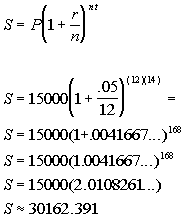*Plug in values shown above into compound form. *Find number inside the (  ) first *Raise the (  )  to the 168th power *Multiply

 So the compound AMOUNT would be \$30162.39 The compound amount is the total amount that is in the account. How do you think we are going to get the interest?? Well we have the principle which is the beginning amount and we have the compound amount which is the end result. Looks like, if we take the difference of the two, that will give us how much interest was earned from beginning to end. What do you think?  Compound amount - principle: 30162.39 - 15000 = 15162.39  So our compound interest is \$15162.39.  Wow, our money doubled and then some - of course it compounded 168 times.Example 7:   Find the a) compound amount AND b) the compound interest for the given investment and rate.  \$20500 for 15 years at an annual rate of 7.5% compounded semiannually.

 P = 20500 r = 7.5% = .075 t = 15 n = semiannually = 2 times a year.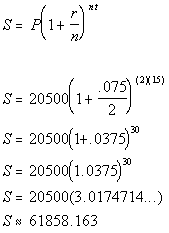*Plug in values shown above into compound form. *Find number inside the (  ) first *Raise the (  )  to the 30th power *Multiply

 So the compound AMOUNT would be \$61858.16 The compound amount is the total amount that is in the account. How do you think we are going to get the interest?? Well we have the principle which is the beginning amount and we have the compound amount which is the end result. Looks like, if we take the difference of the two, that will give us how much interest was earned from beginning to end. What do you think?  Compound amount - principle: 61858.16 - 20500 = 41358.16  So our compound interest is \$41358.16.

 Compound Continuously Formula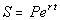where,  S = compound or accumulated amount  P = Principal   (starting value)  r = nominal rate (annual % rate)  t = number of years

 Compounded continuously means that it is compounded at every instant of time.    Note that this formula may look different than the one from your math book.  Sometimes an A is used for compound or accumulated interest instead of an S.  Just keep in mind that no matter how the formula looks the concept is the same.Example 8:   Find the accumulated value of an investment of \$5000 that is compounded continuously for four years at an interest rate of 4.5%.

 P =  5000 r = 4.5% = .045 t = 4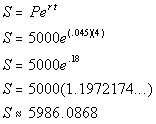*Plug in values shown above into compound form. *Raise e to the 18th power *Multiply

 So the accumulated or compound AMOUNT would be \$5986.09Practice Problems

 These are practice problems to help bring you to the next level.  It will allow you to check and see if you have an understanding of these types of problems. Math works just like anything else, if you want to get good at it, then you need to practice it.  Even the best athletes and musicians had help along the way and lots of practice, practice, practice, to get good at their sport or instrument.  In fact there is no such thing as too much practice. To get the most out of these, you should work the problem out on your own and then check your answer by clicking on the link for the answer/discussion for that  problem.  At the link you will find the answer as well as any steps that went into finding that answer.Practice Problems 1a - 1b:  Approximate the given number using a calculator.  Round to four decimal places.

 1a.(answer/discussion to 1a) 1b.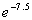(answer/discussion to 1b)Practice Problems 2a - 2b: Graph the given function.

 2a.(answer/discussion to 2a) 2b.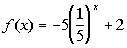(answer/discussion to 2b)Practice Problems 3a - 3b:  Find the a) compound amount AND b) the compound interest for the given investment and rate.

 3a. \$7500 for 25 years at an annual rate of 4.25% compounded monthly.  (answer/discussion to 3a)

 3b. \$3000 for 20 years at an annual rate of 6% compounded quarterly.  (answer/discussion to 3b)Practice Problem 4a: Find the accumulated value for the given investment and rate.

 4a. \$8000 that is compounded continuously for 10 years at an interest rate of 5.5%. (answer/discussion to 4a)Need Extra Help on these Topics?

The following are webpages that can assist you in the topics that were covered on this page.

 http://www.purplemath.com/modules/expofcns.htm This webpage helps you with exponential functions. http://www.purplemath.com/modules/graphexp.htm This webpage goes over graphing exponential functions. http://www.sosmath.com/algebra/logs/log4/log4.html#exponential This website will help you with the definition of and graphing exponential functions.  Stop when it gets to logarithmic functions, we will go over that in another tutorial. http://www.ping.be/~ping1339/exp.htm#Exponential-function This website will help you with the definition of and graphing exponential functions.  Stop when it gets to logarithmic functions, we will go over that in another tutorial.

Go to Get Help Outside the Classroom found in Tutorial 1: How to Succeed in a Math Class for some more suggestions.

Last revised on March 21, 2011 by Kim Seward.